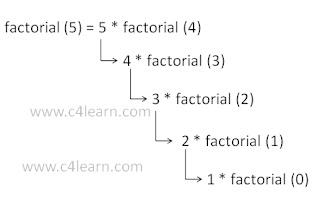## C recursion function

Recursion Concept :

1. Recursion is basic concept in Programming.
2. When Function is defined in terms of itself then it is called as “Recursion Function“.
3. Recursive function is a function which contains a call to itself.
```int factorial(int n){ if(n==0)    return(1); else    return( n * factorial(n-1));}
Warning of using recursive function in C Programming :

Recursive function must have at least one terminating condition that can be satisfied.
Otherwise, the recursive function will call itself repeatably until the run time stack overflows.var disqus_config = function () {
this.page.url = PAGE_URL;  // Replace PAGE_URL with your page's canonical URL variable
this.page.identifier = PAGE_IDENTIFIER; // Replace PAGE_IDENTIFIER with your page's unique identifier variable
};
*/
(function() { // DON'T EDIT BELOW THIS LINE
var d = document, s = d.createElement('script');
s.src = 'https://programmingtutorials.disqus.com/embed.js';
s.setAttribute('data-timestamp', +new Date());
})();

Previous Page

Next Page